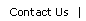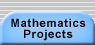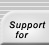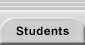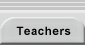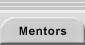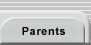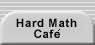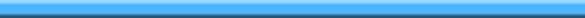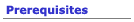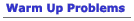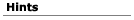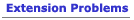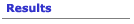## Hints for Postage Stamp Arithmetic

You might want to start with numbers that are smaller than 33 and 20, as in the warm-up problems.  Use postage amounts that your parents and grandparents might remember, like 5- and  7-cent stamps.  Once you understand how to proceed, you can return to the more realistic denominations.

This "hint" isn't really practical for working with actual numbers, but might be a useful "thought experiment."    Write down a list of positive integers, starting with 1, and identify numbers for which you can make postage.  If you identify a certain number of consecutive numbers that can be attained, you can argue that all higher numbers can also be obtained.  Explain how many and why.

You might find it useful to draw a graph with the coordinates being the number of stamps used.  The points with coordinates that are whole numbers -- these are called lattice points -- are the only meaningful points on the graph.  At each lattice point, write the postage amount that is produced, as below.  See if you notice a pattern in the way numbers appear.  The 20- and 33-cent stamp case might be a bit too large unless you use a spreadsheet (see resources) or some other computer program.Some lines like the one drawn above, which is,  pass through a lattice point in the first quadrant; but other don't.  Which ones don't?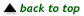Translations of mathematical formulas for web display were created by tex4ht. © Copyright 2003 Education Development Center, Inc. (EDC)